Research Paper

# Analysis of the Tsyganenko Magnetic Field Model Accuracy during Geomagnetic Storm Times Using the GOES Data

Department of Astronomy and Space Science, Chungnam National University, Daejeon 34134, Korea
Corresponding Author Tel: +82-42-821-5463, E-mail: kmin@cnu.ac.kr

Received: Nov 28, 2022; Revised: Dec 8, 2022; Accepted: Dec 12, 2022

## Abstract

Because of the small number of spacecraft available in the Earth’s magnetosphere at any given time, it is not possible to obtain direct measurements of the fundamental quantities, such as the magnetic field and plasma density, with a spatial coverage necessary for studying, global magnetospheric phenomena. In such cases, empirical as well as physics-based models are proven to be extremely valuable. This requires not only having high fidelity and high accuracy models, but also knowing the weakness and strength of such models. In this study, we assess the accuracy of the widely used Tsyganenko magnetic field models, T96, T01, and T04, by comparing the calculated magnetic field with the ones measured in-situ by the GOES satellites during geomagnetically disturbed times. We first set the baseline accuracy of the models from a data-model comparison during the intervals of geomagnetically quiet times. During quiet times, we find that all three models exhibit a systematic error of about 10% in the magnetic field magnitude, while the error in the field vector direction is on average less than 1%. We then assess the model accuracy by a data-model comparison during twelve geomagnetic storm events. We find that the errors in both the magnitude and the direction are well maintained at the quiet-time level throughout the storm phase, except during the main phase of the storms in which the largest error can reach 15% on average, and exceed well over 70% in the worst case. Interestingly, the largest error occurs not at the Dst minimum but 2–3 hours before the minimum. Finally, the T96 model has consistently underperformed compared to the other models, likely due to the lack of computation for the effects of ring current. However, the T96 and T01 models are accurate enough for most of the time except for highly disturbed periods.

Keywords: Tsyganenko magnetic field model; storm time model accuracy; geomagnetic storm

## 1. INTRODUCTION

The magnetosphere is a cylindrical cavity around the Earth that is formed when the solar wind pressure blowing at supersonic speed and the Earth's magnetic field are in equilibrium (Gold 1959). Therefore, the shape of the magnetosphere and various physical phenomena occurring within the magnetosphere are directly influenced by solar activity. Accordingly, it is thought that magnetic field data for all spaces in the magnetosphere will be required when conducting research on the changing magnetosphere. However, due to the difficulty of access to space, there are many difficulties in measuring the desired data for all spaces. As a result, it can explain the phenomenon well and making a model similar to the actual value is an essential factor in studying the global characteristics of the magnetosphere.

Because of this, many researchers are working on creating and developing Earth's magnetosphere model (Tsyganenko 1995, 2000a, b, 2002a, b;Tsyganenko et al. 2003;Tsyganenko & Sitnov 2005, 2007). Since the magnetosphere are directly affected by solar activity, the Earth’s magnetic field must be predicted by assuming these variable factors when calculating the magnetic field model. However, in any calculation, with the variables of simple and constant, the calculation will be easier and produce consistent results. Otherwise, the calculation will be difficult and likely to give inconsistent results. Therefore, it is natural that high accuracy of the model capable of performing complex calculations is required in developing a model similar to the actual value.

As solar activity becomes more active, a geomagnetic storm occurs when the solar wind accompanies the southward interplanetary magnetic field (IMF) due to the occurrence of coronal mass ejections (CMEs) or flares (Lakhina et al. 2004). Moon (2011) showed the correlation between the IMF Bz component and CME-driven storms. During the magnetic storm, a large ring current flowing westward in the equatorial region of the magnetosphere occurs, causing the Earth’s magnetic field to be reduced. Therefore, in this study, when the variables required to calculate the magnetic field model become complicated (when the range of variation is large), we will analyze the accuracy of the model and find out the reliability of the model by comparing the magnetic field model values with the actual observed values (${\stackrel{\to }{B}}_{mod},{\stackrel{\to }{B}}_{obs}$). For this reason, it was decided that the period of intense solar activity was selected for the analysis when the variables for the calculation of the magnetic field model show complex behavior. Hence, the periods of the magnetic storm were examined. The magnetic field model calculation during the event period used Tsyganenko’s 04 model, 01 model, and 1996 model (hereafter T04, T01, T96 model) among the most commonly used empirical models. The T96 has an clearly defined magnetopause, large-scale Region 1 and 2 Birkeland current systems, and the IMF penetration across the boundary. The T01 represents the variable configuration of the inner magnetosphere for different IMF conditions and ground Dst index (Tsyganenko 2002a, b). Lastly, the T04 is a dynamical model of the storm-time geomagnetic field in the inner magnetosphere, using space magnetometer data taken during several major events in 1996–2000 and simultaneous observations of the solar wind and IMF variance (Tsyganenko & Sitnov 2005).

These magnetic field models are provided by the Geopack module of Space Physics Environment Data Analysis Software (SPEDAS) which is an IDL open source data analysis tool. The Tsyganenko magnetic field model is a tool that can calculate ${\stackrel{\to }{B}}_{mod}$ based on information such as the H component of geomagnetic field change, IMF value, solar wind number density, solar wind velocity, etc. By using this, based on the comparison of ${\stackrel{\to }{B}}_{mod}$ (magnetic field value calculated by model) and ${\stackrel{\to }{B}}_{obs}$ (magnetic field value measured by satellite) during the period without an event (when solar activity is quiet), the trend is analyzed to find out the consistency in the accuracy of the model. Eventually, the accuracy of the model during the event is quantitatively analyzed.

## 2. DATA AND METHODS

2.1 DATA Preparation

Firstly, in order to identify the period when the magnetic storm event occurred and the quiet period, the Dst index data provided by Kyoto University for the events from 1990 to 2016 were examined, and among the events which show the Dst index –70 nT or less in the main phase due to the magnetic storm. Among them, events such as Fig. 1, which shows changes due to storm sudden commencements (Park et al. 2015) and smooth recovery phase (Lee et al. 2001), were selected. As a result, a total of 12 events were selected (Table 1).Fig. 1. Typical Sym-H index component behavior during the magnetic storm (January 11–17, 2000). ① Initial phase, ② main phase, ③ recovery phase.
Table 1. List of magnetic storm events and satellites used for the analysis of magnetic field model data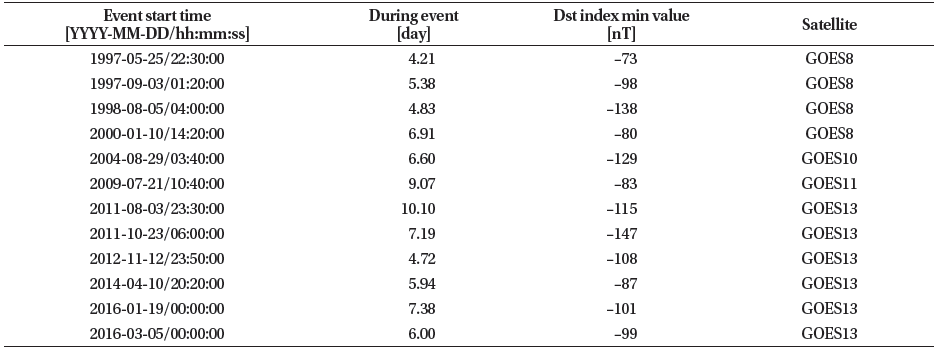2.2 Reference Magnetic Field Data (GOES Satellite)

Next, we need ${\stackrel{\to }{B}}_{obs}$ to compare to the calculated ${\stackrel{\to }{B}}_{mod}$ to evaluate the accuracy. For this data, among many satellites operating in the magnetosphere, magnetic field data obtained from satellites of stationary orbit with a fixed distance from the center of the Earth were investigated. ${\stackrel{\to }{B}}_{obs}$ data were obtained from the GOES satellite which already had magnetic field observation data for the 12 event periods selected above, and the data were converted into Geocentric Solar Magnetic coordinates for this study.

2.3 Tsyganenko Magnetic Field Model Data

The variables required to calculate the Tsyganenko magnetic field model are obtained from OMNI data provided by NASA. OMNI is a multi-source dataset of near- Earth solar wind magnetic fields and plasma parameters from November 1963 to today, and has been updated regularly with new data (Papitashvili & King 2006). OMNI provides the IMF (magnitude and vector), flow velocity (magnitude and vector), flow pressure, proton density, and several additional parameters including sunspot and geomagnetic indices and energetic proton fluxes from IMP and GOES. To compare this calculated ${\stackrel{\to }{B}}_{mod}$ with ${\stackrel{\to }{B}}_{obs}$, GOES position data of the same time zone as ${\stackrel{\to }{B}}_{obs}$ was also used as a variable. Through this, it was worked to compare the actual observation value and the model value of the magnetic field at the same time and space.

2.4 Error Analysis

First, based on the data mentioned above, the Dst index and the dynamic pressure of the solar wind were compared together for the 12 magnetic storm events, and considering the correlation between the magnetic storm and the dynamic pressure, ${\stackrel{\to }{B}}_{obs}$ and ${\stackrel{\to }{B}}_{mod}$ were shown together and compared (Fig. 2).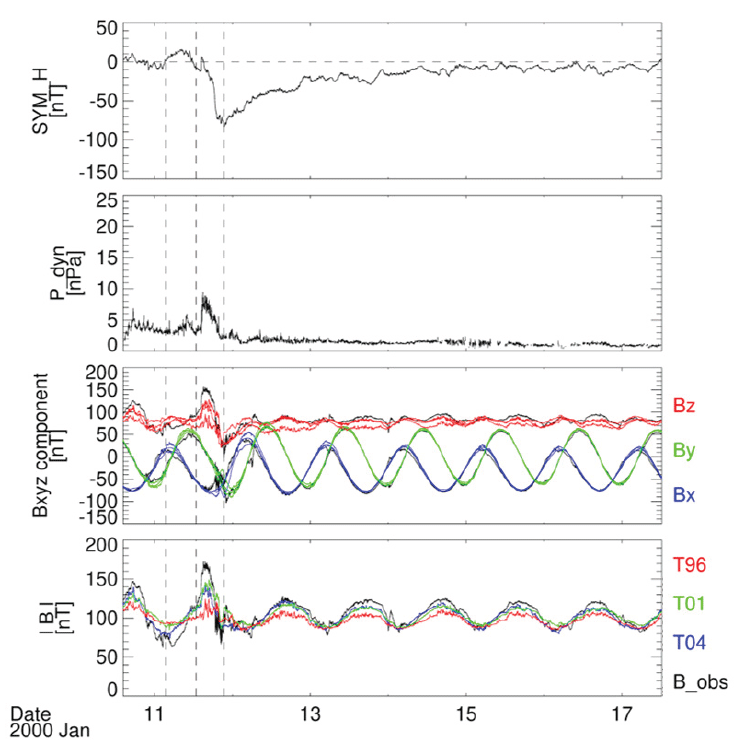Fig. 2. Comparison of observed values and model values during magnetic storm events. From above, Sym-H index, solar wind dynamic pressure, observed magnetic field (black) and model magnetic field for each components (x is blue, y is green, and z is red; Different lines for each color show the values of different models, and it is only to check which components show differences from the observed values, and it does not indicate which model each line is), and finally observed magnetic field magnitude (black) and calculated magnetic field for each model (T96 is red, T01 is green, and T04 is blue). T96, Tsyganenko’s 1996 model; T01, Tsyganenko’s 01 model; T04, Tsyganenko’s 04 model.

The Dst index provided by Kyoto University and Sym-H index of OMNI data provided by NASA represent the same physical phenomenon. While the Dst index is an hourly average index, Sym-H provides a one-minute average index. Thus, in order to show high resolution, Sym-H is used instead of Dst index in this study.

Since the 12 magnetic storm events have different minimum values of the Dst index and different periods of initial, main, and recovery phases, it is necessary to relatively match these events to analyze data. Hence, for each event, the time of the point where the Dst index is minimum was set as the zero point, and the data were reset to the time when the magnetic storm progressed, and the error of ${\stackrel{\to }{B}}_{mod}$ for each corresponding time was calculated. Also, at this time, since the magnetic field data is a vector value, not only the strength of the magnetic field but also the error in direction was considered. According to the following equation, the relative error (${|\stackrel{\to }{B}|}_{err}$) and directional error (θerr) were calculated.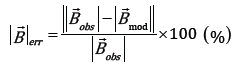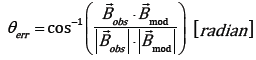Based on the errors obtained through this process, the error of the model during the magnetic storm event period for each magnetic field model was analyzed quantitatively through superposed epoch analysis.

## 3. RESULTS

Superposed epoch analysis was performed to show how the error rate changes before and after the magnetic storm by overlapping errors about each the magnetic field models. In this analysis, the time of the main phase, in which the Dst index is the smallest, was set as 0h.

As can be seen from Fig. 3, the T04 model maintained an error of 5% before and after storm, and then showed an error rate of 13% at the peak. In the case of the T01 model, it was also confirmed that the error rate was maintained at around 5%–6% and then the error rate was slightly less than 15% at peak. And the T96 model showed an error rate of less than 10% before and after storm, showing a larger overall error rate than the more recent version of the magnetic field model. Among the three models, the latest version of the T04 model is the most suitable to obtain model data for the intensity of the magnetic field using the Tsyganenko magnetic field model.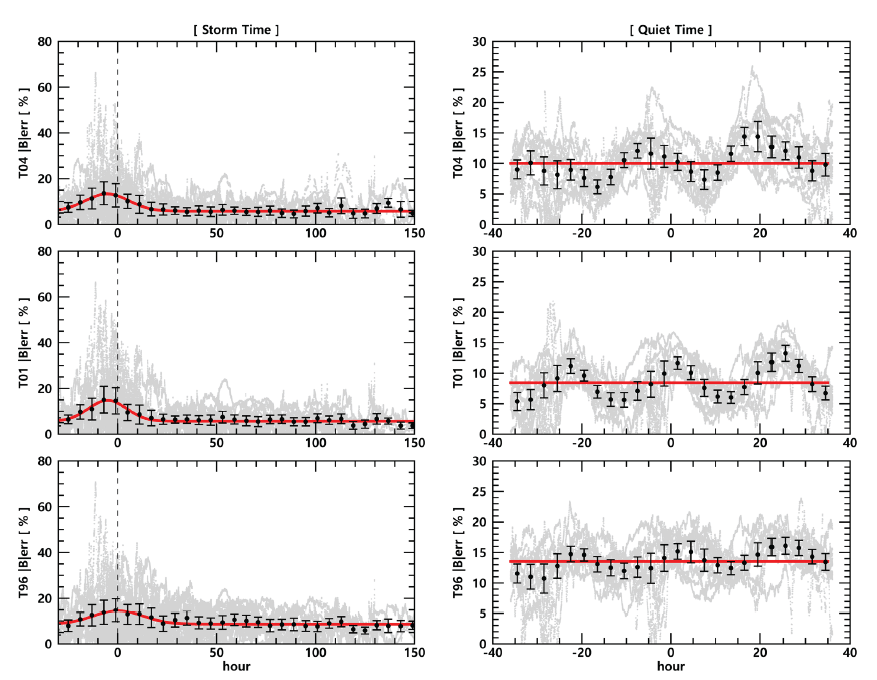Fig. 3. The relative error for the magnetic field magnitude of each model during the geomagnetic storm time (left) and the quiet time (right) (Result of T04, T01, and T96 model from above). T04, Tsyganenko’s 04 model; T01, Tsyganenko’s 01 model; T96, Tsyganenko’s 1996 model.

The three figures on the right side of Fig. 3 are the error rates of the magnetic field models during periods of quiet solar activity. Unexpectedly, T01 showed the best performance with an average error of 8%. In addition, it seems that each model has an error rate of about 8%–14%, and considering this, it is thought that the effect of the magnetic storm period on each model will actually be around 10%.

Similar results can be obtained with errors in the direction of the magnetic field model (Fig. 4). When the error is at its peak, it can be seen that the direction of ${\stackrel{\to }{B}}_{mod}$ differs from ${\stackrel{\to }{B}}_{obs}$ by 0.2–0.4 radian (π/15 – π/8). Since cosθ ≈ 1 – θ2/2, the error in the direction is θ2 and can be said to be within 15%.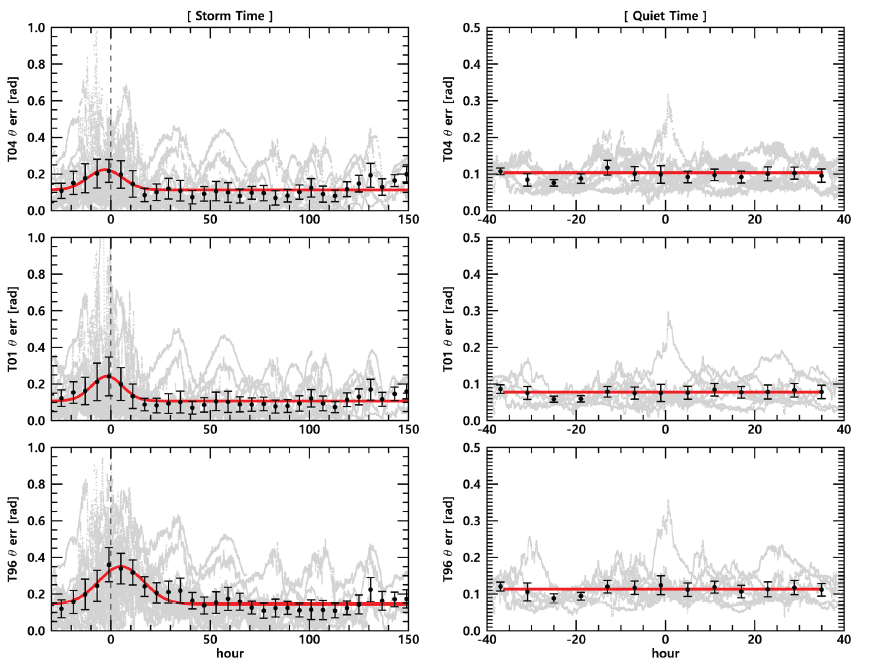Fig. 4. Direction error for each model's magnetic field direction during the geomagnetic storm period (left) and during the quiet period (right) (Result of T04, T01, and T96 model from above). T04, Tsyganenko’s 04 model; T01, Tsyganenko’s 01 model; T96, Tsyganenko’s 1996 model.

Only parts where the errors of magnitude and direction exceeded 10% and 0.1rad were separately extracted, and a distribution in which errors were large for each magnetic local time (MLT) was confirmed. The gray pie-chart in Fig. 5 corresponds to the normalized value obtained by dividing the number of data with an error of more than 10% from the data at each MLT point (bin size 10°). In the green piechart, the ${|\stackrel{\to }{B}|}_{err}$ of grater than 10% was averaged for each point. In T04 and T01, day-night asymmetry was seen on the nightside rather than on the dayside. On the contrary, T96 were more on the dayside, as well as more data with large errors in all spaces than the previous two models. The large number of data errors of more than 10% on the dayside of T96 will be mentioned in Section 4. Looking at the green pie-chart, the error rate seems to be similar in all spaces, but in T96, it was confirmed that the error rate increased by nearly 20% on the Earth’s night side, that is, in the magnetosphere tail area.Fig. 5. Normalized number of data (gray) and averaged relative error (green) of grate than 10% in each model with magnetic local time. T04, Tsyganenko’s 04 model; T01, Tsyganenko’s 01 model; T96, Tsyganenko’s 1996 model.

The day-night asymmetry of the above mentioned model error is more pronounced in the direction error (Fig. 6). The average error is also lower on the day side at T04 and T01, and it may be seen that it is evenly distributed overall at T96. Taken together, T01 seems a little more appropriate to obtain directional information of the magnetic field for the dayside during the geomagnetic storm, and it would be helpful to use the latest model for the magnitude. It will also be possible to show the least error in using T01 in quiet time.Fig. 6. Normalized number of data (gray) and averaged direction error (green) of grate than 0.1 radian in each model with magnetic local time. T04, Tsyganenko’s 04 model; T01, Tsyganenko’s 01 model; T96, Tsyganenko’s 1996 model.

## 4. DISCUSSION

Overall, the errors in these model values are believed to come from the error in the process of calculating the value of ${\stackrel{\to }{B}}_{mod,z}$ using the Tsyganenko magnetic field model or the lack of data necessary for calculating the magnetic field model. As can be seen in the comparison of the x, y, z components of ${\stackrel{\to }{B}}_{obs}$ and ${\stackrel{\to }{B}}_{mod}$ in Fig. 2, the x, y components of ${\stackrel{\to }{B}}_{obs}$ and ${\stackrel{\to }{B}}_{mod}$ do not differ significantly from each other. In contrast, the z component shows a noticeable difference compared to the other components and generally shows a tendency of ${\stackrel{\to }{B}}_{obs,z}>{\stackrel{\to }{B}}_{mod,z}$, which was observed not only during magnetic storm periods but also during periods of quiet solar activity (Fig. 7).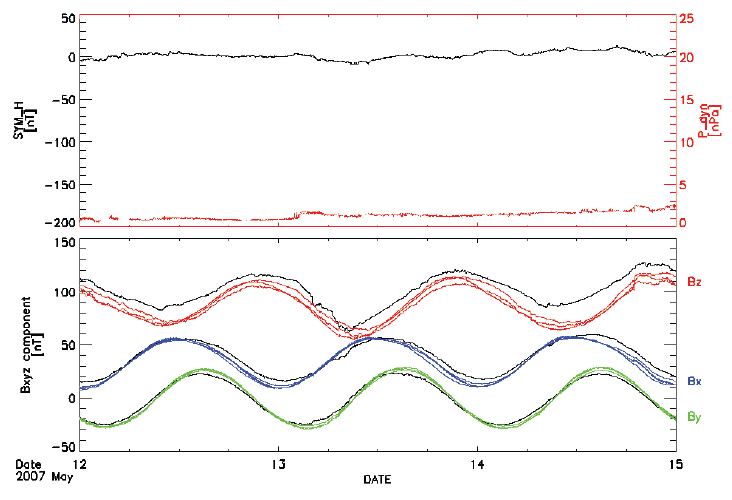Fig. 7.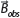(below, black) and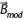(below, color classification for each component) during periods of quiet solar activity; Different lines for each color show the values of different models, and it is only to check which components show differences from the observed values, and it does not indicate which model each line is.

It is speculated that the difference in the z component caused errors in the direction and strength of the magnetic field model. A more accurate magnetic field model is expected to be calculated if we resolve this issue.

Also, as shown in Figs. 3 and 4, when the errors of each magnetic field model are at their peak, the time of peak turned out to be slightly to the left of 0h, rather than 0h (the point where the Dst index is the minimum). That is, the error value tended to increase during the main phase of the magnetic storm period. Referring to Fig. 2 as a representative example, ${\stackrel{\to }{B}}_{obs}$ shows a rapid change at the two points where the dynamic pressure suddenly increases, and the Dst index is the minimum. While ${\stackrel{\to }{B}}_{mod}$ is similar to ${\stackrel{\to }{B}}_{obs}$ to some extent when the Dst index is minimum, the difference between ${\stackrel{\to }{B}}_{obs}$,z and ${\stackrel{\to }{B}}_{mod,z}$ obviously increased when the dynamic pressure rapidly increased. In other words, it can be considered that dynamic pressure has a significant impact on the magnetic field data.

The dynamic pressure is given by the following equation.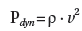• ρ: solar wind density, υ: solar wind speed

Further study is needed to determine the reason why magnetic field data respond sensitively to dynamic pressure: solar wind density or solar wind speed.

Finally, comparing the results of each model, T04 and T01 show similar results, while T96 shows a significant difference. We believe that this is mainly because of the simple empirical ring current model used in T96; subsequent models use more accurate approximations by calculating ring current based on the electric current considering an observed distribution of particle pressure, anisotropy and dawn-dusk asymmetry of ring current (Tsyganenko 2002a). However, in the case of the T04 model, the effect of partial ring current cannot be ignored in the situation where the solar wind dynamic pressure is increasing (Park et al. 2011). In addition, post-T96 models were developed by combining the effects of azimuthally asymmetric ring current and fieldaligned current. For this reason, the performance of the T96 seems to be lower than that of the subsequent models, and the use of the T96 model should be avoided at least during storm time.

## 5. SUMMARY

In order to analyze the accuracy of the Tsyganenko magnetic field models (T04, T01, and T96) on the prediction of change in the geomagnetic field due to magnetic storms, 12 magnetic storm events from 1990 to 2016 were selected. Calculations were performed for the magnetic field models, and a comparison of the results with the actual observed values was made. The errors for the magnetic field magnitude and direction were obtained for each magnetic field model, then the errors for each magnetic field model were analyzed by superposed epoch analysis. Although the newer models generally expect better performance, our results suggest that it may be appropriate to use previous versions of the model depending on the situation. During the geomagnetic storm, the use of the T04 model, which has an average error of about 13% even at the point where the error is the largest, would be effective. During the quiet time, surprisingly, T01 showed the best performance with an average error of 8%. In addition, by identifying points with spatially large errors during storm time, it quantitatively grasps the increase in errors in the magnetotail.

## ACKNOWLEDGMENTS

This work was supported by the research fund of Chungnam National University.

## References

1.

Gold T, Motions in the magnetosphere of the Earth, J. Geophys. Res. 64, 1219-1224 (1959).2.

Lakhina GS, Alex S, Tsurutani BT, Gonzalez WD, Research on historical records of geomagnetic storms, Proc. Int. Astron. Union 2004, 3-15 (2004).3.

Lee DY, Hwang JA, Min KW, Lee ES, Cho KS, et al., A statistical study of magnetic storm recovery phase: preliminary results, J. Astron. Space Sci. 18, 27-32 (2001).4.

Moon GH, Variation of magnetic field (By, Bz) polarity and statistical analysis of solar wind parameters during the magnetic storm period, J. Astron. Space Sci. 28, 123-132 (2011).5.

Papitashvili NE, King JH, A draft high resolution OMNI data set, Proceedings of the 2006 AGU Fall Meetings, San Francisco, CA, 10-15 Dec 2006.6.

Park JS, Kim KH, Lee DH, Lee ES, Jin H, Geosynchronous magnetic field response to solar wind dynamic pressure, J. Astron. Space Sci. 28, 27-36 (2011).7.

Park W, Lee J, Yi Y, Ssessanga N, Oh S, Storm sudden commencements without interplanetary shocks, J. Astron. Space Sci. 32, 181- 187 (2015).8.

Tsyganenko NA, Modeling the Earth’s magnetospheric magnetic field confined within a realistic magnetopause, J. Geophys. Res. Space Phys. 100, 5599-5612 (1995).9.

Tsyganenko NA, Modeling the inner magnetosphere: the asymmetric ring current and Region 2 Birkeland currents revisited, J. Geophys. Res. Space Phys. 105, 27739-27754 (2000a).10.

Tsyganenko NA, Solar wind control of the tail lobe magnetic field as deduced from Geotail, AMPTE/IRM, and ISEE 2 data, J. Geophys. Res. Space Phys. 105, 5517-5528 (2000b).11.

Tsyganenko NA, A model of the near magnetosphere with a dawn-dusk asymmetry 1. Mathematical structure, J. Geophys. Res. Space Phys. 107, SMP 12-11-SMP 12-15 (2002a).12.

Tsyganenko NA, A model of the near magnetosphere with a dawn-dusk asymmetry 2. Parameterization and fitting to observations, J. Geophys. Res. Space Phys. 107, SMP 10-11- SMP 10-17 (2002b).13.

Tsyganenko NA, Singer HJ, Kasper JC, Storm-time distortion of the inner magnetosphere: how severe can it get?, J. Geophys. Res. Space Phys. 108, 1209 (2003).14.

Tsyganenko NA, Sitnov MI, Modeling the dynamics of the inner magnetosphere during strong geomagnetic storms, J. Geophys. Res. Space Phys. 110, A03208 (2005).15.

Tsyganenko NA, Sitnov MI, Magnetospheric configurations from a high-resolution data-based magnetic field model, J. Geophys. Res. Space Phys. 112, A06225 (2007).